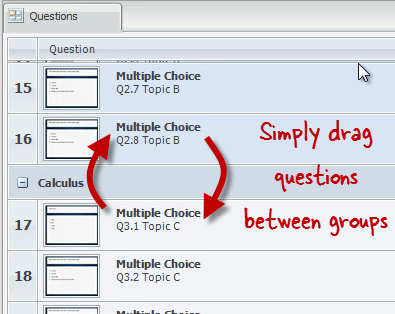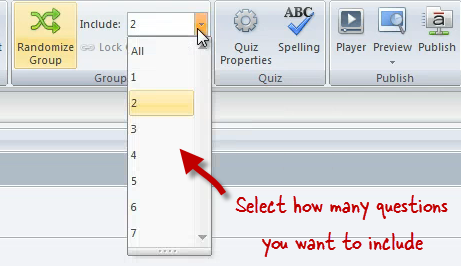In our previous tutorial we learned how to create freeform questions. In this tutorial, we will see how Articulate Quizmaker's question group feature lets you organize your questions into groups and randomize your quizzes so that each learner sees a different set of questions.

Watch this video first, then follow the steps below to practice.

## Practice Activity

In this exercise, we will create two new question groups, rename them and move questions between groups. In the end you will have 24 questions divided among three question groups with 10 random questions included in your quiz from the pool of 24.Rename the existing question group to "Algebra"

• Add two new question groups. Name one "Geometry" and the other "Calculus"
• Move all of the Topic C questions into the Calculus Question Group (TIP: Use the CTRL key to select multiple questions.)
• Move all of the Topic B questions into the Geometry Question GroupNext we will set up our quiz to include a random selection of questions from each group.

• Randomize all three groups to include 4 questions from the Algebra group, 3 questions from the Geometry group and 3 questions from the Calculus group.Finally, we'll lock two related questions together so that they will always appear together in the correct order in your quiz.

• Lock the fourth and fifth questions in the Algebra group with each other so they will always be displayed together in the right order.
• Lock question Q3.3 Topic C to the top of the group so it will always be the first question from that group to appear in your quiz.

That's all there is to it! When you publish, for any group you've randomized, users will see the questions in a random order—but any locked questions will be shown in the position you've specified. And if you've chosen a sample size for any question group, users will see only that number of questions from each group.

In the next tutorial, we'll see how to adjust the Quizmaker player properties.